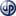Weights and Measures

Cooking Measurements

1 teaspoon = 1/6 fl. ounce 1 Tablespoon = 1/2 fl. ounce 1 tablespoon = 3 teaspoons
1 dessert spoon (UK) = 2.4 teaspoons 16 tablespoons = 1 cup 12 tablespoons = 3/4 cup
10 tablespoons + 2 teaspoons = 2/3 cup 8 tablespoons = 1/2 cup 6 tablespoons = 3/8 cup
5 tablespoons + 1 teaspoon = 1/3 cup 4 tablespoons = 1/4 cup 2 tablespoons = 1/8 cup
2 tablespoons + 2 teaspoons = 1/6 cup 1 tablespoon = 1/16 cup 2 cups = 1 pint
2 pints = 1 quart 3 teaspoons = 1 tablespoon 48 teaspoons = 1 cup
1 cup = 8 fluid ounces 2 cups= 1 pint 2 cups= 16 fluid ounces
1 quart = 2 pints 4 cups = 1 quart 4 cups = 32 fluid ounces
8 cups = 4 pints 8 cups = 1/2 gallon 8 cups = 64 fluid ounces
4 quarts =1 gallon 4 quarts = 128 fluid ounces 1 gallon (gal) = 4 quarts
16 ounces = 1 pound Pinch = Less than 1/8 teaspoon

F to C Degrees Conversion Chart

225F = 110C = Gas mark 1/4
250F = 120C = Gas mark 1/2
275F = 140C = Gas mark 1
300F = 150C = Gas mark 2
325F = 160C = Gas mark 3
350F = 180C = Gas mark 4
375F = 190C = Gas mark 5
400F = 200C = Gas mark 6
425F = 220C = Gas mark 7
450F = 230C = Gas mark 8
475F = 240C = Gas mark 9
500F = 260C
550F = 290C

Imperial to Metric

1/4 teaspoon = 1.25 ml 1/2 tsp = 2.5 ml 1 tsp = 5 ml
1 tablespoon = 15 ml 1/4 cup = 60 ml 1/3 cup = 75 ml
1/2 cup = 125 ml 2/3 cup = 150 ml 3/4 cup = 175 ml
1 cup = 250 ml 1 1/8 cups = 275 ml 1 1/4 cups = 300 ml
1 1/2 cups = 350 ml 1 2/3 cups = 400 ml 1 3/4 cups = 450 ml
2 cups = 500 ml 2 1/2 cups = 600 ml 3 cups = 750 ml
3 2/3 cups = 900 ml 4 cups = 1 liter

Weight Conversion
1/2 oz = 15g 1 oz = 25 g 2 oz = 50 g
3 oz = 75 g 4 oz = 100 g 6 oz = 175 g
7 oz = 200 g 8 oz = 250 g 9 oz = 275 g
10 oz = 300 g 12 oz = 350 g 1 lb = 500 g
1 1/2 = 750 g 2 lb = 1 kg

Bar Drink Measurements

1 dash = 6 drops
3 teaspoons = 1/2 ounce
1 pony = 1 ounce
1 jigger = 1 1/2 ounce
1 large jigger = 2 ounces
1 std. whiskey glass = 2 ounces
1 pint = 16 fluid ounces
1 fifth = 25.6 fluid ounces
1 quart = 32 fluid ounces

Cake Pan Size Conversions
20cm springform cake pan = 8 inch
20cm square cake pan = 8 inch
23cm springform cake pan = 9 inch
25cm springform cake pan = 10 inchCopyright secured by Digiprove © 2016 Yvonne  Oots